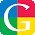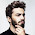# EMF Equation of a Generator

Let φ =flux/pole in weber.
Z=total number of armature conductors = No. of slots * No.of conductors/slot.
P = No. of poles.
A = No. of parallel paths in armature.
N = armature rotation in rpm.
E = EMF induced in any parallel path in armature.
Generated EMF = e.m.f. generated in one of the parallel paths.
Average EMF generated/conductor = (dφ/dt) volt.

Now, flux cut/conductor in one revolution, dφ = (φ*P) web.
Number of revolution per second = N/60;
Then, time for one revolution, dt = 60/N second.
Hence according to Faraday's laws of electromagnetic induction,

EMF generated/conductor= (dφ/dt) = (φZPN/120) Volt.

#### For a wave-wound generator

No. of parallel paths is 2
No. of conductors (in series) in one path = Z/2

#### For a lap-wound generator

No. of parallel paths = P
No. of conductors (in series) in one path = Z/P

Then, EMF generated/path = [(φPN/60)*(Z/P)] = (φZN/60) Volt.
In general, generated EMF = [(φPN/60)*(Z/A)]= (φZPN/60A) Volt

Where    A=2 for wave-winding.
A= P for lap-winding.4 CommentsComments

#### Read Comment Policy We have Zero Tolerance to Spam. Chessy Comments and Comments with Links will be deleted immediately upon our review.

1.very well written information on E.M.F. Equation of D.C. Generator thank you sir

2.While trying to guarantee that exchange gear is introduced by code, some nearby government offices require that the installer get an allow preceding establishment. A qualified circuit repairman will know when an allow is required, and how to approach acquiring one.Generator Review

3.Do you have chronic headaches and can't find the cause for them. Have you been to doctor after doctor and tried one medication after another without much luck? If so, Electromagnetic Fields (EMFs) may be the unsuspecting culprit.emf protection

4.Since math is unquestionably no a piece of cake, it's imperative that understudies stay aware of exercises by putting aside time to rehearse ordinary. In the event that understudies prop up at it, the issues will get simpler as the arrangement bode well. step-by-step solution